# Lecture 4 Work Electric Potential and Potential Energy

• Slides: 47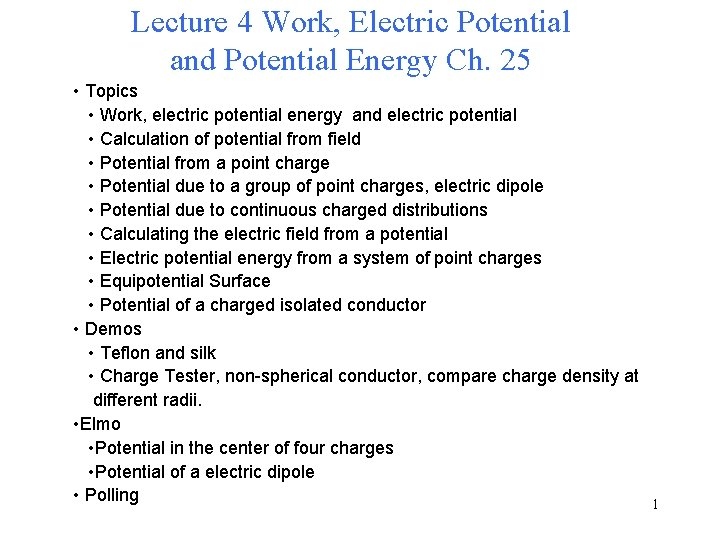Lecture 4 Work, Electric Potential and Potential Energy Ch. 25 • Topics • Work, electric potential energy and electric potential • Calculation of potential from field • Potential from a point charge • Potential due to a group of point charges, electric dipole • Potential due to continuous charged distributions • Calculating the electric field from a potential • Electric potential energy from a system of point charges • Equipotential Surface • Potential of a charged isolated conductor • Demos • Teflon and silk • Charge Tester, non-spherical conductor, compare charge density at different radii. • Elmo • Potential in the center of four charges • Potential of a electric dipole • Polling 1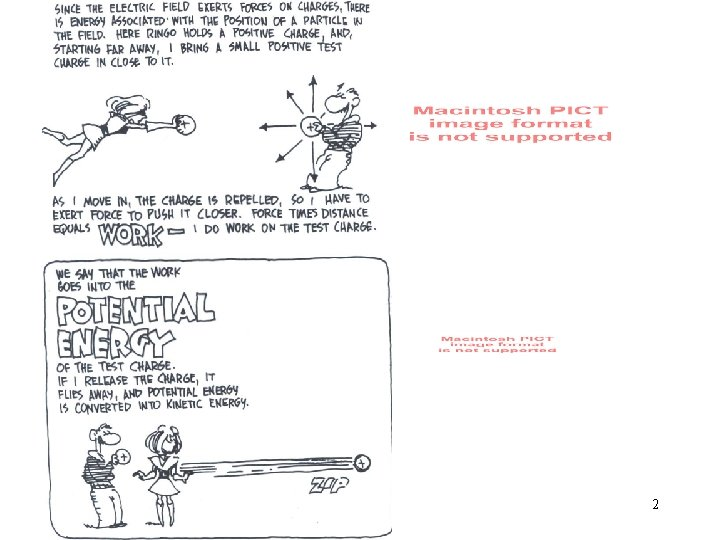2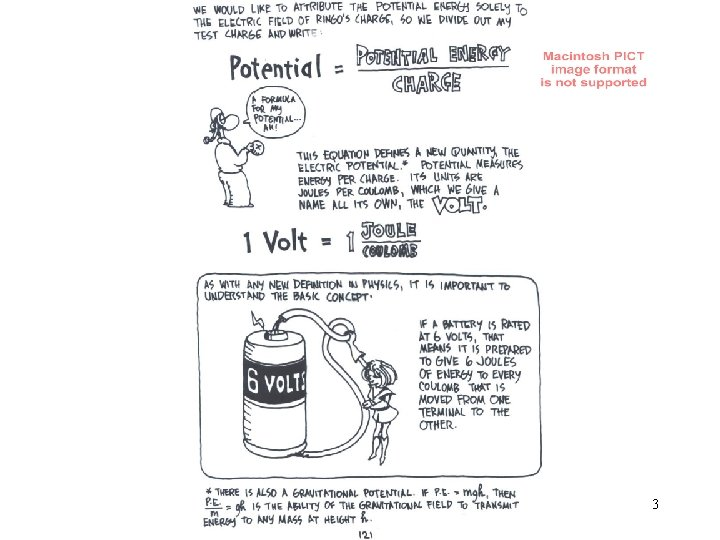3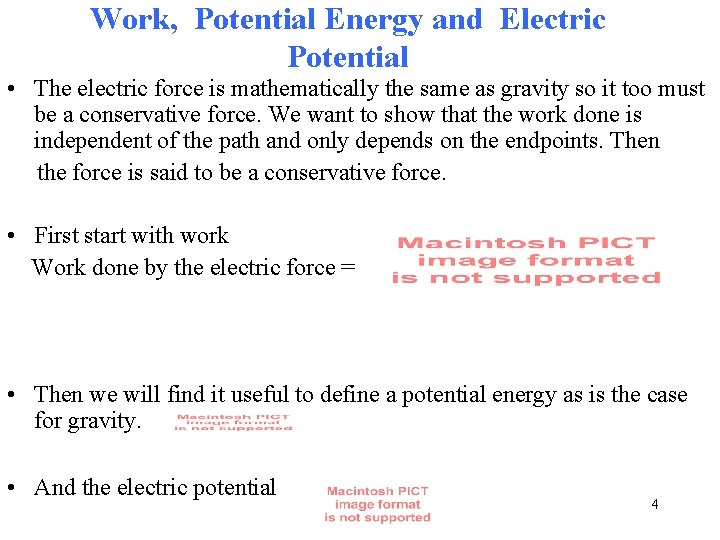Work, Potential Energy and Electric Potential • The electric force is mathematically the same as gravity so it too must be a conservative force. We want to show that the work done is independent of the path and only depends on the endpoints. Then the force is said to be a conservative force. • First start with work Work done by the electric force = • Then we will find it useful to define a potential energy as is the case for gravity. • And the electric potential 4Lets start with a uniform electric field and find the work done for a positive test charge. P 2 a b P 3 c E P 1 5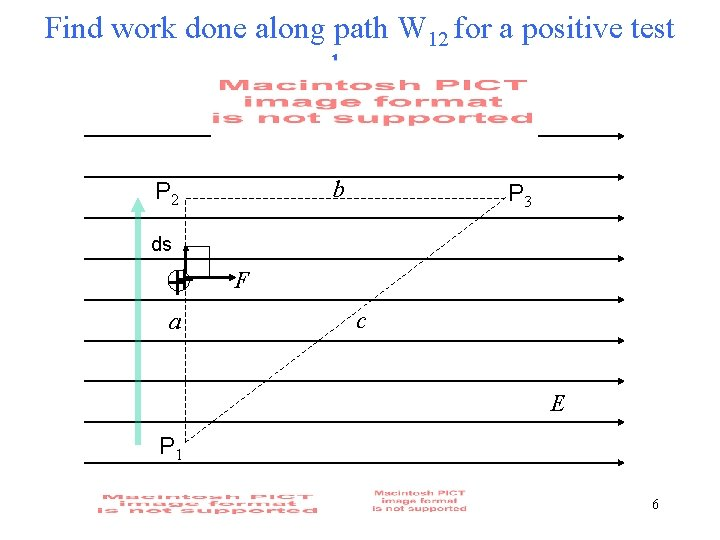Find work done along path W 12 for a positive test charge b P 2 P 3 ds F a c E P 1 6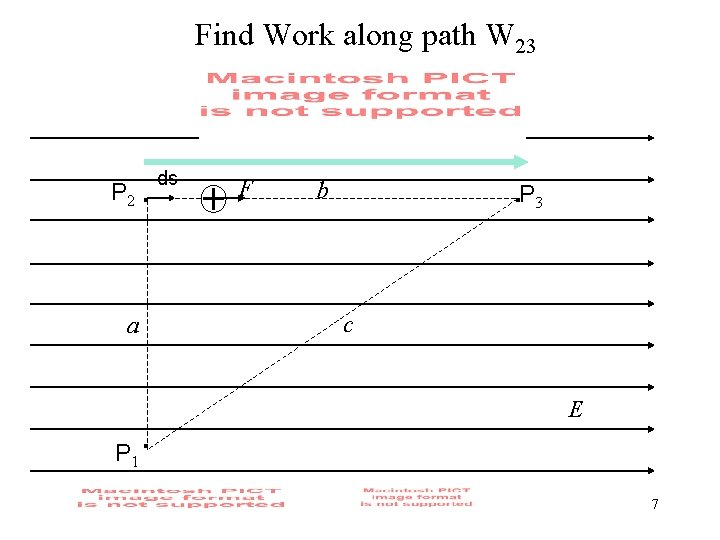Find Work along path W 23 P 2 a ds F b P 3 c E P 1 7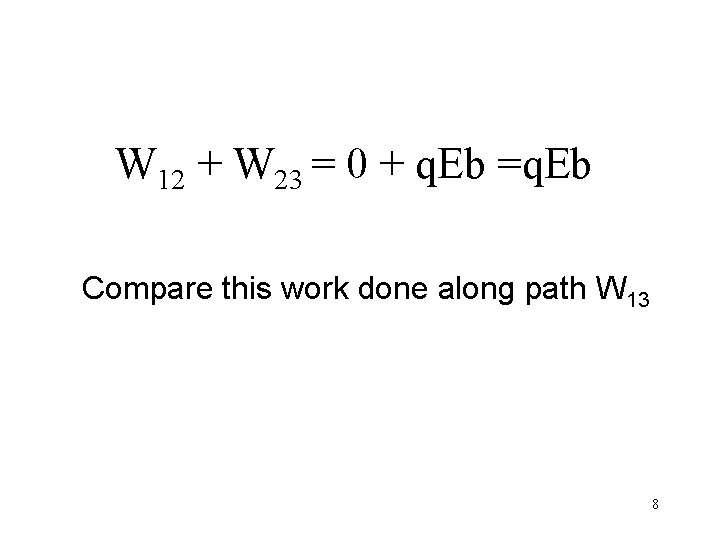W 12 + W 23 = 0 + q. Eb =q. Eb Compare this work done along path W 13 8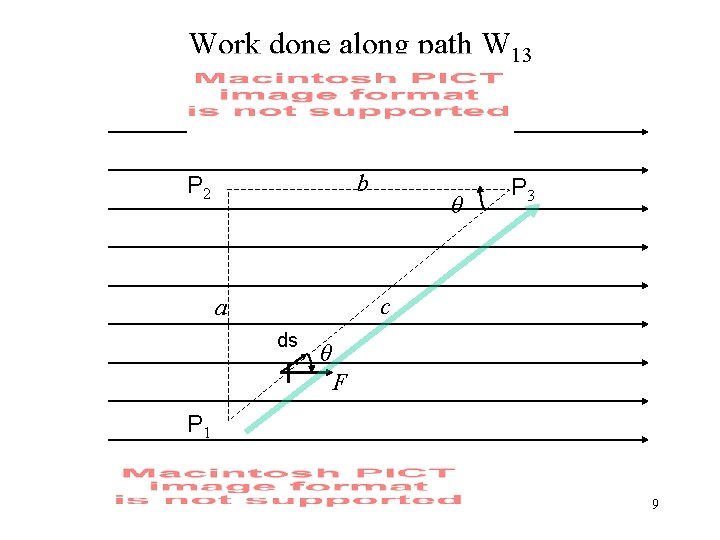Work done along path W 13 b P 2 θ P 3 c a ds θ F P 1 9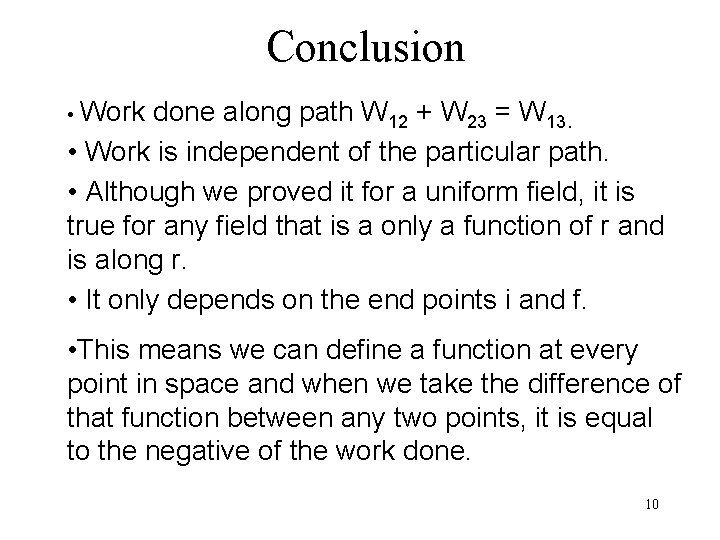Conclusion • Work done along path W 12 + W 23 = W 13. • Work is independent of the particular path. • Although we proved it for a uniform field, it is true for any field that is a only a function of r and is along r. • It only depends on the end points i and f. • This means we can define a function at every point in space and when we take the difference of that function between any two points, it is equal to the negative of the work done. 10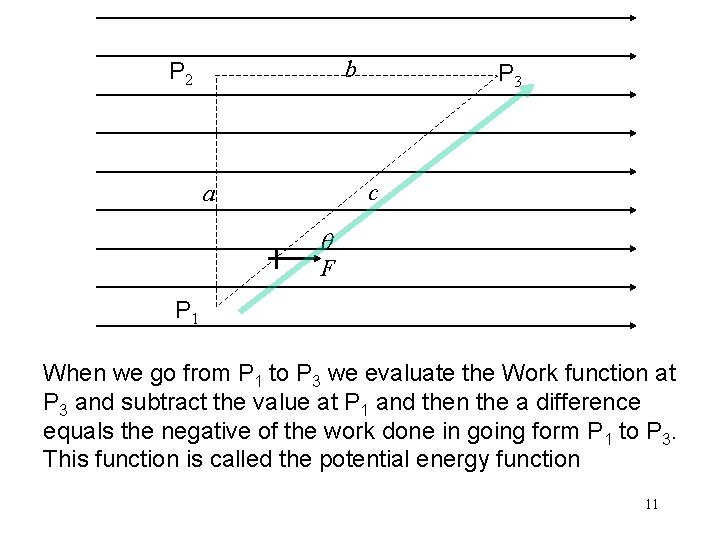b P 2 P 3 c a F P 1 When we go from P 1 to P 3 we evaluate the Work function at P 3 and subtract the value at P 1 and then the a difference equals the negative of the work done in going form P 1 to P 3. This function is called the potential energy function 11Example of finding the Potential Energy Function U in a Uniform Field What is the electric potential difference for a unit positive charge moving in an uniform electric field from a to b? E E d a b If we set the origin at xb = 0, and measure from b to a, then Ub=0 and the potential energy function is U=q. Ed This is analogy with gravitation where we U =mgh. 12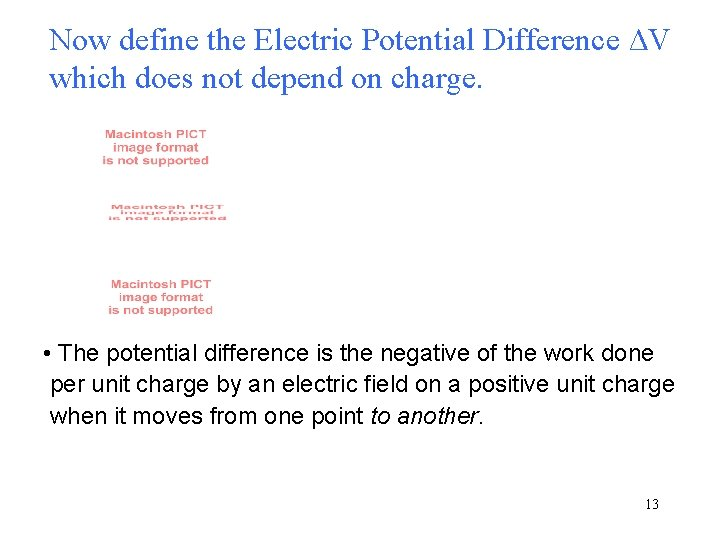Now define the Electric Potential Difference ΔV which does not depend on charge. • The potential difference is the negative of the work done per unit charge by an electric field on a positive unit charge when it moves from one point to another. 13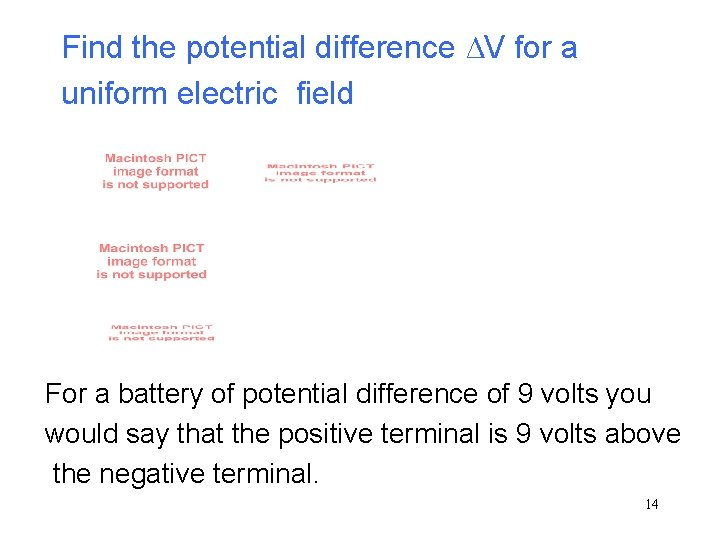Find the potential difference ΔV for a uniform electric field For a battery of potential difference of 9 volts you would say that the positive terminal is 9 volts above the negative terminal. 14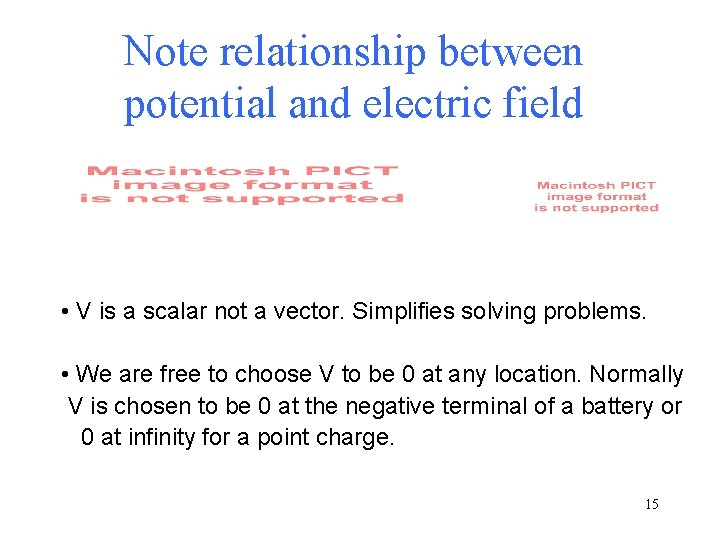Note relationship between potential and electric field • V is a scalar not a vector. Simplifies solving problems. • We are free to choose V to be 0 at any location. Normally V is chosen to be 0 at the negative terminal of a battery or 0 at infinity for a point charge. 15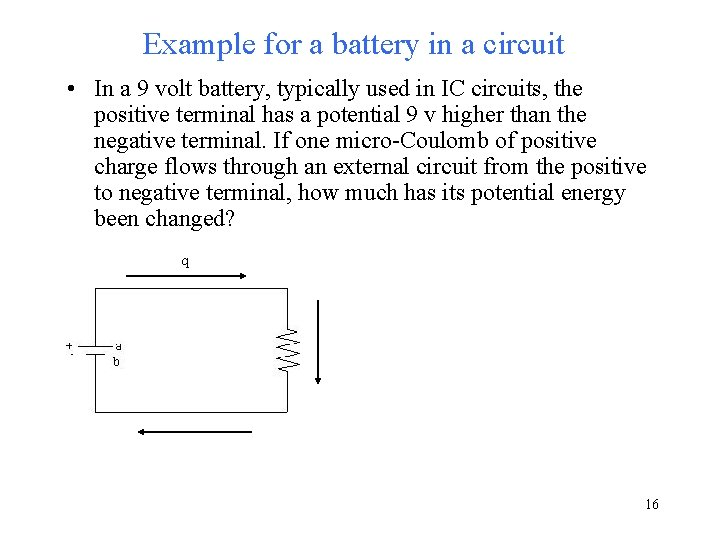Example for a battery in a circuit • In a 9 volt battery, typically used in IC circuits, the positive terminal has a potential 9 v higher than the negative terminal. If one micro-Coulomb of positive charge flows through an external circuit from the positive to negative terminal, how much has its potential energy been changed? q 16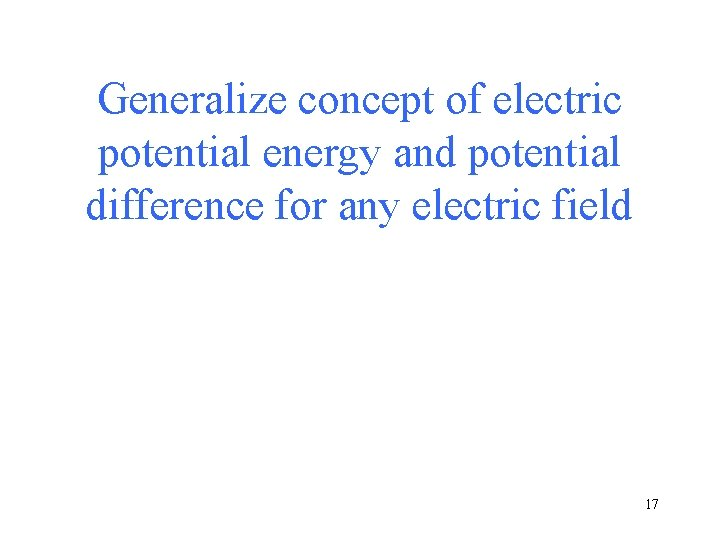Generalize concept of electric potential energy and potential difference for any electric field 17= - Work done by the electric force = y x (independent of path, ds) Therefore, electric force is a conservative force. 18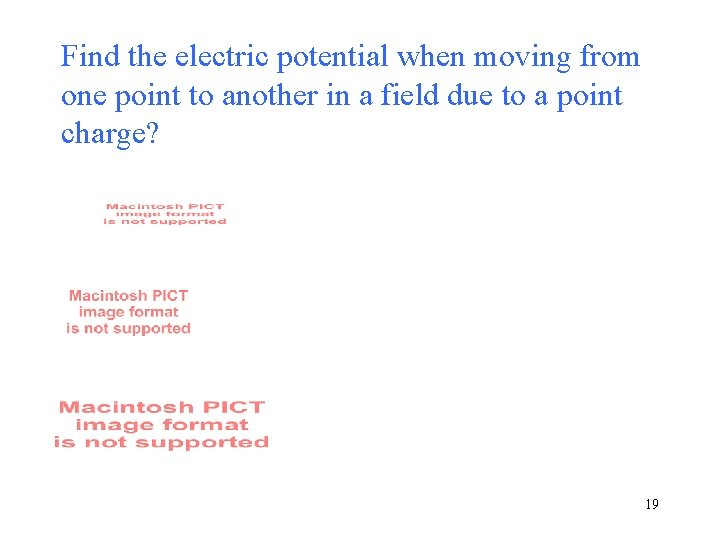Find the electric potential when moving from one point to another in a field due to a point charge? 19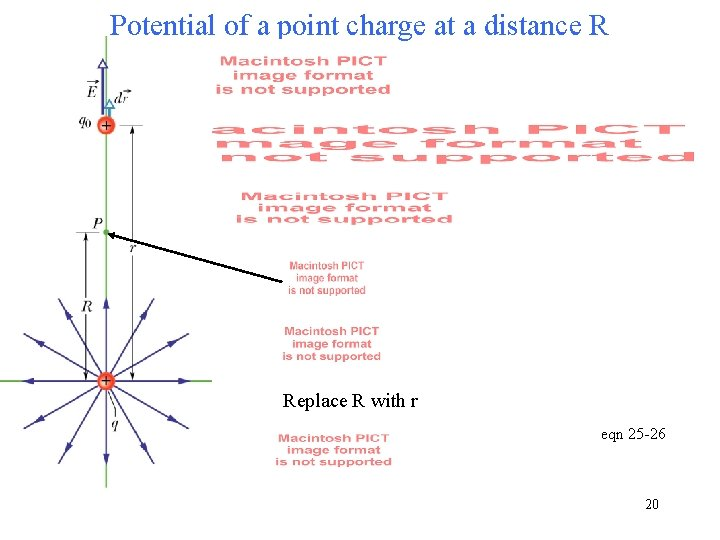Potential of a point charge at a distance R Replace R with r eqn 25 -26 20Electric potential for a positive point charge • V is a scalar • V is positive for positive charges, negative for negative charges. • r is always positive. • For many point charges, the potential at a point in space is the simple algebraic sum (Not a vector sum) 21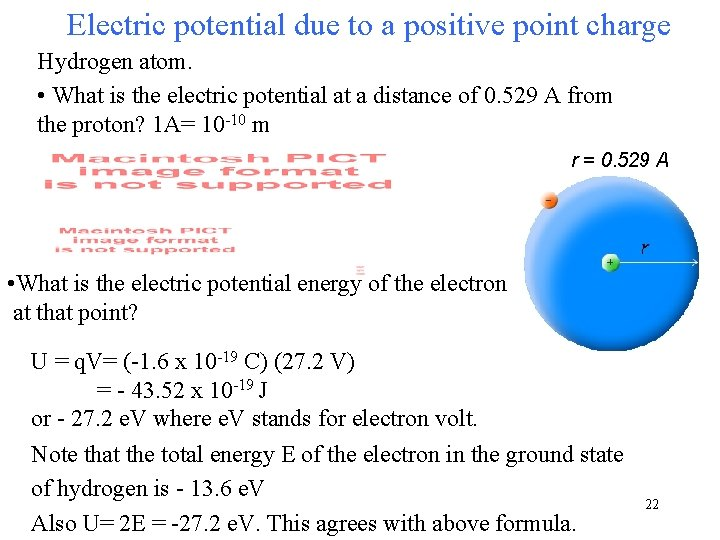Electric potential due to a positive point charge Hydrogen atom. • What is the electric potential at a distance of 0. 529 A from the proton? 1 A= 10 -10 m r = 0. 529 A • What is the electric potential energy of the electron at that point? U = q. V= (-1. 6 x 10 -19 C) (27. 2 V) = - 43. 52 x 10 -19 J or - 27. 2 e. V where e. V stands for electron volt. Note that the total energy E of the electron in the ground state of hydrogen is - 13. 6 e. V Also U= 2 E = -27. 2 e. V. This agrees with above formula. 22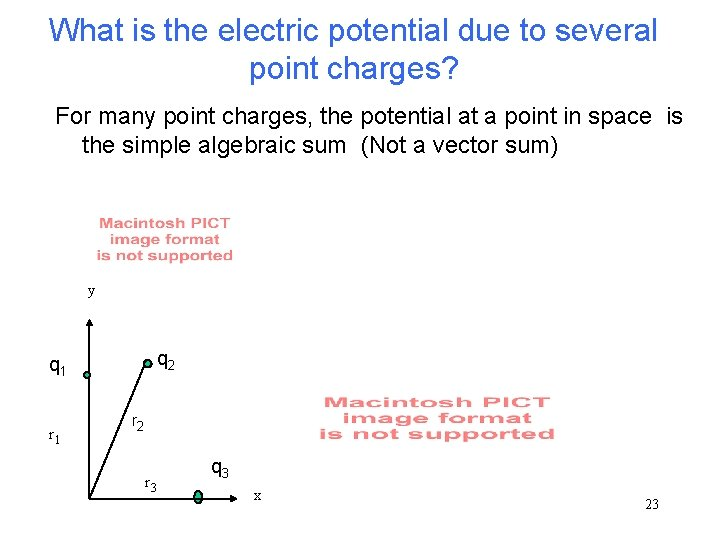What is the electric potential due to several point charges? For many point charges, the potential at a point in space is the simple algebraic sum (Not a vector sum) y q 2 q 1 r 2 r 3 q 3 x 23Four Point charges 24Two point charges that are opposite and equal What is the potential due to a dipole? 25Potential for a Continuous Distribution of Charge Point charge For an element of charge Integrate V 26Chaper 24 Problem 22. With V = 0 at infinity, what is the electric potential at P, the center of curvature of the uniformly charged nonconducting rod? 27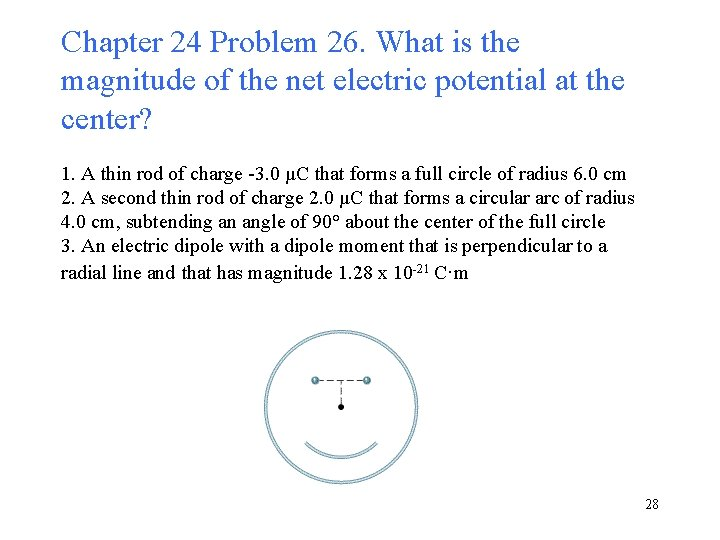Chapter 24 Problem 26. What is the magnitude of the net electric potential at the center? 1. A thin rod of charge -3. 0 µC that forms a full circle of radius 6. 0 cm 2. A second thin rod of charge 2. 0 µC that forms a circular arc of radius 4. 0 cm, subtending an angle of 90° about the center of the full circle 3. An electric dipole with a dipole moment that is perpendicular to a radial line and that has magnitude 1. 28 x 10 -21 C·m 2829Figure 24 -44 shows a thin plastic rod of length L and uniform positive charge Q lying on an x axis. With V = 0 at infinity, find the electric potential at point P 1 on the axis, at distance d from one end of the rod. 3031Potential due to a ring of charge • Direct integration. Since V is a scalar, it is easier to evaluate V than E. • Find V on the axis of a ring of total charge Q. Use the formula for a point charge, but replace q with elemental charge dq and integrate. Point charge For an element of charge r is a constant as we integrate. V This is simpler than finding E because V is not a vector. 32Potential due to a line charge We know that for an element of charge dq the potential is For the line charge let the charge density be l. Then dq=ldx Now, we can find the total potential V produced by the rod at point P by integrating along the length of the rod from x=0 to x=L 33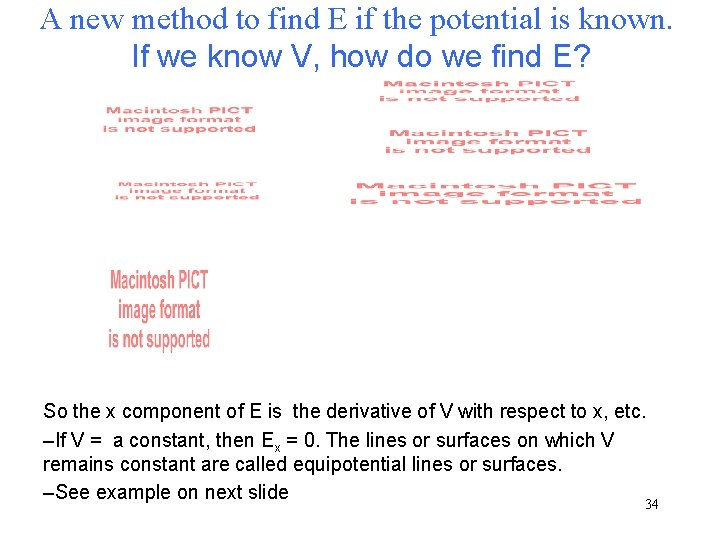A new method to find E if the potential is known. If we know V, how do we find E? So the x component of E is the derivative of V with respect to x, etc. –If V = a constant, then Ex = 0. The lines or surfaces on which V remains constant are called equipotential lines or surfaces. –See example on next slide 34Equipotential Surfaces • Three examples • What is the equipotential surface and equipotential volume for an arbitrary shaped charged conductor? • See physlet 9. 3. 2 Which equipotential surfaces fit the field lines? 35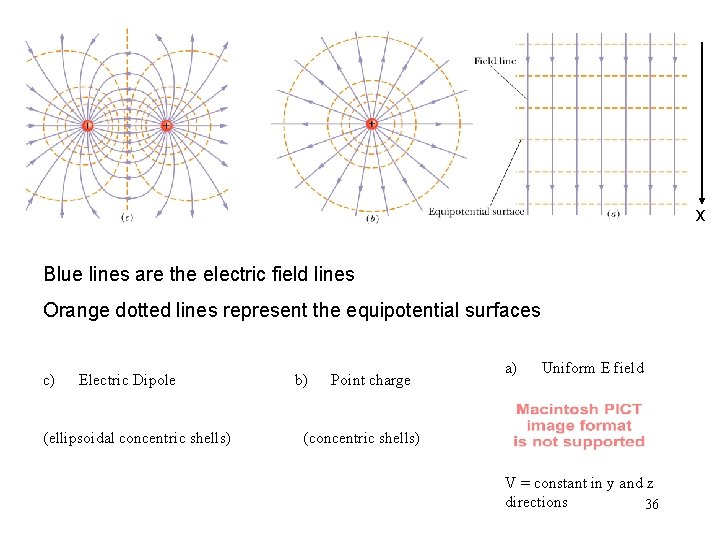x Blue lines are the electric field lines Orange dotted lines represent the equipotential surfaces c) Electric Dipole (ellipsoidal concentric shells) b) Point charge a) Uniform E field (concentric shells) V = constant in y and z directions 36Electric Potential Energy U of a system of charges How much work is required to set up the arrangement of Figure 24 -46 if q = 3. 20 p. C, a = 54. 0 cm, and the particles are initially infinitely far apart and at rest? q 1 q 4 q 2 W=U q 3 37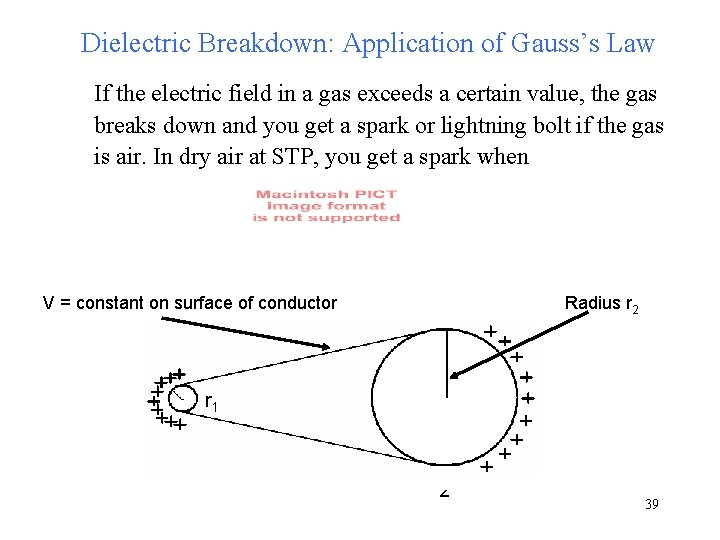Dielectric Breakdown: Application of Gauss’s Law If the electric field in a gas exceeds a certain value, the gas breaks down and you get a spark or lightning bolt if the gas is air. In dry air at STP, you get a spark when V = constant on surface of conductor Radius r 2 r 1 1 2 39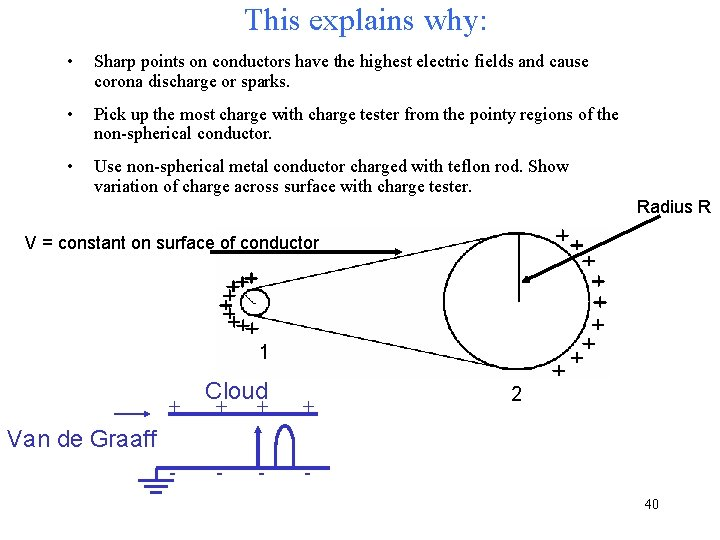This explains why: • Sharp points on conductors have the highest electric fields and cause corona discharge or sparks. • Pick up the most charge with charge tester from the pointy regions of the non-spherical conductor. • Use non-spherical metal conductor charged with teflon rod. Show variation of charge across surface with charge tester. Radius R V = constant on surface of conductor 1 + Cloud + + + - - - 2 Van de Graaff - 40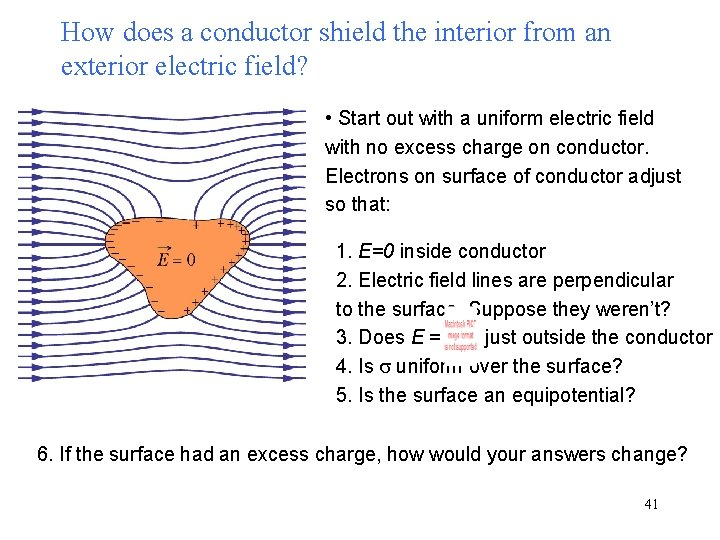How does a conductor shield the interior from an exterior electric field? • Start out with a uniform electric field with no excess charge on conductor. Electrons on surface of conductor adjust so that: 1. E=0 inside conductor 2. Electric field lines are perpendicular to the surface. Suppose they weren’t? 3. Does E = just outside the conductor 4. Is s uniform over the surface? 5. Is the surface an equipotential? 6. If the surface had an excess charge, how would your answers change? 41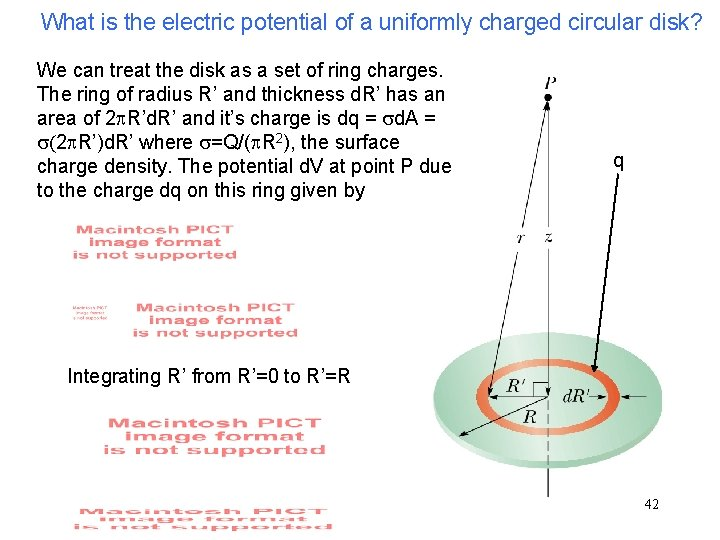What is the electric potential of a uniformly charged circular disk? We can treat the disk as a set of ring charges. The ring of radius R’ and thickness d. R’ has an area of 2 p. R’d. R’ and it’s charge is dq = sd. A = s(2 p. R’)d. R’ where s=Q/(p. R 2), the surface charge density. The potential d. V at point P due to the charge dq on this ring given by q Integrating R’ from R’=0 to R’=R 42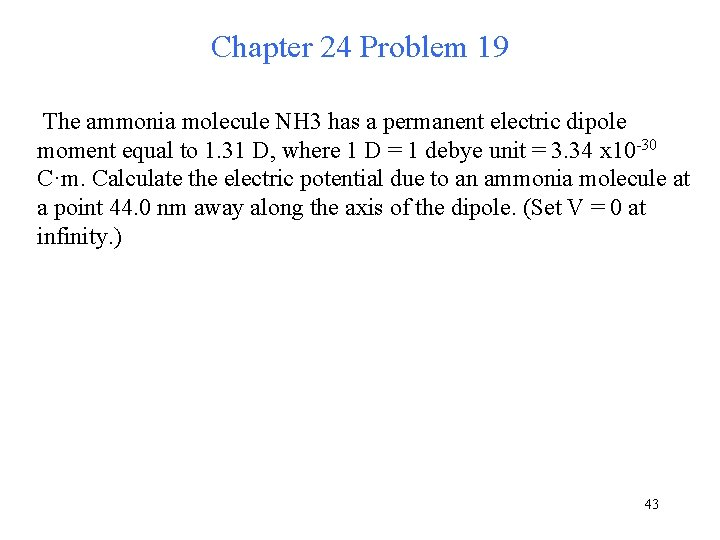Chapter 24 Problem 19 The ammonia molecule NH 3 has a permanent electric dipole moment equal to 1. 31 D, where 1 D = 1 debye unit = 3. 34 x 10 -30 C·m. Calculate the electric potential due to an ammonia molecule at a point 44. 0 nm away along the axis of the dipole. (Set V = 0 at infinity. ) 43Chapter 24 Problem 55 Two metal spheres, each of radius 1. 0 cm, have a center-to-center separation of 2. 2 m. Sphere 1 has charge +2. 0 x 10 -8 C. Sphere 2 has charge of -3. 8 x 10 -8 C. Assume that the separation is large enough for us to assume that the charge on each sphere is uniformly distributed (the spheres do not affect each other). Take V = 0 at infinity. (a) Calculate the potential at the point halfway between the centers. (b) Calculate the potential on the surface of sphere 1. (c) Calculate the potential on the surface of sphere 2. 4445Chapter 24 Problem 57 A metal sphere of radius 11 cm has a net charge of 2. 0 x 10 -8 C. (a) What is the electric field at the sphere's surface? (b) If V = 0 at infinity, what is the electric potential at the sphere's surface? (c) At what distance from the sphere's surface has the electric potential decreased by 500 V? 4647